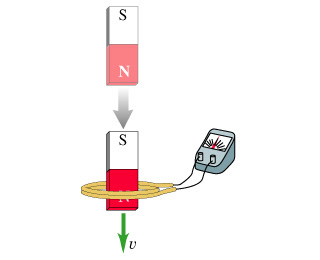# Problem: When a magnet is plunged into a coil at speed v, as shown in the figure, a voltage is induced in the coil and a current flows in the circuit.If the speed of the magnet is doubled, the induced voltage is ________ .a.) twice as greatb.) four times as greatc.) half as greatd.) unchanged

###### FREE Expert Solution

The induced emf in the coil is given by Faraday's law of electromagnetic induction:

$\overline{){{\mathbf{\epsilon }}}_{\mathbf{i}\mathbf{n}\mathbf{d}}{\mathbf{=}}{\mathbf{B}}{\mathbf{l}}{\mathbf{v}}}$, where B is the magnetic field, l is length, and v is speed.

84% (346 ratings)###### Problem Details

When a magnet is plunged into a coil at speed v, as shown in the figure, a voltage is induced in the coil and a current flows in the circuit.If the speed of the magnet is doubled, the induced voltage is ________ .

a.) twice as great

b.) four times as great

c.) half as great

d.) unchanged